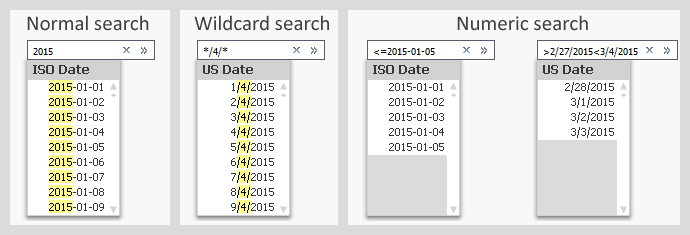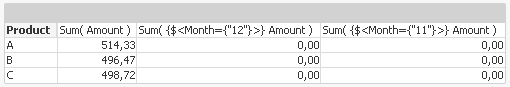# Qlik Design Blog

All about product and Qlik solutions: scripting, data modeling, visual design, extensions, best practices, etc.

Announcements
QlikWorld 2022, LIVE in Denver CO., May 16-19, 2022. REGISTER NOW TO RECEIVE EARLY BIRD PRICINGEmployee

## Dates in Set Analysis

Several aspects of the Qlik search mechanism has been described in previous posts. There is however one that has not been covered: Search in dual fields, e.g. dates. This post will try to explain the basics.

When making searches in text fields, you can search either by using a normal search or by using a wildcard search, and when you search in numeric fields you can use a numeric search. But what about dual fields, like dates, where you have both a textual and a numeric representation?

The answer is displayed in the picture below.Normal searches and wildcard searches are straightforward and need not be explained. Numeric searches are also possible and do pretty much what you expect them to.

You should however note that the search string in a numeric search must contain the correct formatted date. It is in most cases not possible to use the numeric value of the date. E.g. you cannot search for 42005 when you want Jan 1st 2015, even though this is the value of the date.

The same logic is used in Set Analysis, which means that a correct Set Analysis expression with a date could look like this:

Sum( {\$<Date={"<=2015-02-28"}>} Amount)

Often you want the Set Analysis expression to be dynamic, and then you need to put a dollar expansion with an aggregation function inside it. One case is that you want to compare the selected month with the preceding month. In principal, the solution is something similar to the following:

Selected (Last possible) month:     Sum( {\$<Month={"\$(=Max(Month))"}>}  Amount )
2nd Last month:                                    Sum( {\$<Month={"\$(=Max(Month)-1)"}>}  Amount )

The Max(Month) will calculate the last possible month, and the dollar expansion will enter this value into the expression before the expression is parsed.

How the expression looks after the dollar expansion can be seen in the column header of a QlikView table. The above formulas have been used in the table below. Note that the dollar expansions with Max(Month) have been replaced with numbers.So far, so good.

However, the above formulas will not work. First, if you have created the Month using the Month() function, the field is cyclic which means that December of one year has a higher numeric value than January the following year, although it comes before January. Hence, the Max() function will not respect the order of months belonging to different years.

Secondly, the Month field has a dual value. This means that the Max(Month) will return a numeric when you need the textual value (‘Dec’) in the Set analysis expression.

One solution is to use a sequential month instead, and format it the same way everywhere:

Script:

Date(MonthStart(Date),'MMM-YY') as Month,

Expressions:

Sum( {\$<Month={"\$(=Date(Max(Month),'MMM-YY'))"}>} Amount )

Here the field Month is a date - the first day of the month - but formatted with just month and year. In other words: A number that equals roughly 42000 and is formatted as ‘Jan-15’. The same formatting is applied inside the dollar expansion. Note the column headers below.Often it is practical to put the calculation of the Set analysis condition in variables. This way, the formula is kept in one place only and the Set analysis expressions become simpler and easier to read:

Script:

Set vLastMonth=      "=Date(Max(Month),'MMM-YY')";
Date(MonthStart(Date),'MMM-YY') as Month,

Expressions:

Sum( {\$<Month={"\$(vLastMonth)"}>} Amount )
Sum( {\$<Month={"\$(v2ndLastMonth)"}>} Amount )

Note that the variable definitions start with equals signs. This way they will be recalculated at every click.

Summary: Format the dates used inside Set analysis expressions, and use variables to simplify the expressions.

HIC

Further reading related to this topic:

The Search String

Data Types in QlikView

Cyclic or Sequential?

A Primer on Set Analysis